# Elastic and Inelastic Electron-Nucleus Scattering Form Factors for Be9 Nucleus

Department of Physics, College of Science, University of Sulaimani, Sulaymaniyah, Kurdistan Region, Iraq

Received: 05-04-2020 Accepted: 13-08-2020 Published: 17-08-2020
DOI: 10.21928/uhdjst.v4n2y2020.pp56-62

ABSTRACT

The computations of the elastic and inelastic Coulomb form factors for the electron-nucleus scattering of beryllium nucleus Be9 have performed with core polarization (CP) effects including the realistic Michigan sum of three range Yukawa (M3Y) interaction and the other residual interaction which is modified surface delta interaction (MSDI). In the calculations, root mean square charge density and charge radii include for the ground states. The perturbation theory is adopted to compute the CP using the harmonic oscillators potential to calculate single-particle radial wave functions. The comparison has been done between the theoretical calculations of Coulomb form factors by MSDI interaction, realistic M3Y interaction, and the experimental results that measured by other workers, it noticed that the Coulomb form factors for the (M3Y) interaction gave a reasonable depiction of the measured data.

Index Terms: Beryllium Nucleus, Core Polarization Effect, Electron Scattering, Form Factor, Harmonic Oscillator

### 1. INTRODUCTION

There are two essential reasons why electron-nucleus scattering is such a successful apparatus used for studying nuclear structure. The primary one belongs to the reality that the main interaction occurring between the electron and the nucleus is well known . The origin of the second reason that makes electron scattering is a valuable method in examining the properties of nuclear structure comes from its ability to identify the excited states, spins, and parities, through the calculations of the reduced matrix elements of nuclear transitions. Basically, in the electron scattering with a relatively weak interaction, the interactions of the electron with charge and the nuclear current density occur where they described by the theory of quantum electrodynamics .

One of the great (standard) effective interactions for light nuclei is the Cohen-Kurath , for 1p-shell (1p1/2, 1p3/2) nuclei with core 2He4. In addition, different macroscopic and microscopic theories have been used to analyze excitation states in Be nucleus. The form factor calculations were done by utilizing the model space (MS) wave functions alone which were not sufficient for duplicating the experimental data of the electron-nucleus scattering . Therefore, the electron scattering Coulomb form factors in the p-shell nucleus (Be9) have been investigated by taking into account higher energy configurations outside the p-shell MS which are named core polarization effects .

Many research studies have focused their efforts on the improvement and development of the electron scattering. Starting with Hofstadter who was the primary to utilize high-energy electron beams given by the Stanford linear electron accelerator to discover electron scattering and an old work of Sir Nevill Mott which was used electrons against point nuclei in his experiment as the relativistic scattering of Dirac particles. Then, he established a series formulation for the cross-section of the elastic scattering, also he allowed to estimating formula , .

Elastic and inelastic electron scattering for the light nuclei using Born approximation had performed by Uberall and Ugincius . In the last decades, the single-particle quadrupole transitions of Coulomb electron-nucleus scattering form factors studied in the B10 which is the p-shell nucleus by Majeed , whereas the studies included a microscopic theory in the core polarization (CP) effects for the excitation states up to 2ℏw by employing the modified surface delta interaction (MSDI).

The charge density distributions and charge radii of the nucleus were distinguished from the investigation of elastic electron scattering data . However, Sharrad et al.  have used the charge density distributions of the ground state for determining the Coulomb form factors using the approximation rule which is the plane wave Born approximation with the two-body short range correlation. Consequently, Radhi et al.  presented inelastic Coulomb and electromagnetic form factors for F19 in each positive parity and negative parity states by applying the single-particle states shell model and Hartree–Fock method.

At present, Raheem et al.  have been calculated the elastic Coulomb C0 form factors for a few sd-shell nuclei using nucleon-nucleon effective interaction, which is two-body (Michigan sum of three range Yukawa [M3Y]) as residual interactions with considering the CP matrix elements.

This work is devoted to calculate the theoretical Coulomb electron scattering form factors for Be9 by considering the role of the MS besides the CP effects using MSDI and the realistic interaction named M3Y including root mean square charge density along with charge radii for the ground states. The harmonic oscillator (HO) wave function will be adopted as a single particle wave function. To do this, first needed to use shell model code (OXBASH) to calculate the one-body density matrix (OBDM) elements , .

Finally, the theoretical calculations of Coulomb form factors by MSDI, M3Y interactions are compared with the experimental results.

### 2.1. Coulomb Form Factor

The Coulomb electron scattering form factors of a given multipolarity (J) is a function of transfer momentum (q) and it can be described in term of reduced matrix elements (in spin state) of the transition operator :

Where, Ji and Jf are, respectively, the initial and final total angular momentum, while Z is the number of proton (atomic number), TJ(q) is the multipole operator of electron scattering, and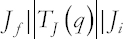is the reduced many body matrix element.

The best description of the experimental form factors requires to correct the form factor in Equation (1) corresponding to the center of mass correction and the finite size correction of the nucleon :

Here, b is the HO size parameter that obtained from the experiment, A is the nuclear mass number, and the final term in the above equation is the correction coefficient. The reduced matrix element in Equation (1) can be expressed in two terms, the first one is MS term and the other is CP term .

The MS reduced matrix element in the spin and isospin spaces of the transition operator TJ is performed as the sum of the product of the (OBDM) elements which are in neutron-proton formalism OBDM(β, α ,J, τz, i, f) multiplied by the single-particle reduced matrix elements as follow :

In addition, the CP reduced matrix element can be represented as:

Where, α and β are, respectively, the initial and final single-particle states for the MS when isospin included, the index τz is the third component of nucleons Pauli isospin which used to identify the nucleons with τz = 1, −1 for protons and neutrons, respectively, and the OBDM determined macroscopically for the elastic scattering by the initial and final nuclear wave functions, while it obtained from OXBASH code for inelastic scattering. The single-particle matrix element is determined from:

Where,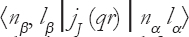is the radial part matrix element of the spherical Bessel function jJ (qr) which is calculated in [19, Equation (23)] of our published article; and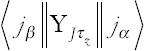represents the reduced matrix element of the spherical Harmonics Yz.

The single-particle matrix element can represent according to the first-order perturbation theory as :

The single-particle matrix element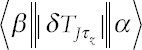in the above equation obtained from the particle hole excitation with the first-order perturbation including residual interaction (V) for the MSDI and M3Y interaction .

Here, Ho is the unperturbed Hamiltonian, Ea, Eb are the initial and final states of energy, the Q operator projects the outside space of the MS, both the indices a1 and a2, are, respectively, run over particle and hole states,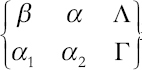is the six-j symbol and e is the single-particle energy. Every matrix element in the Equation (7) is obtained in iso-scalar (T = 0) and isovector (T = 1) formalism with Λ = JT and Γ=J’ T’.

The single-particle energies are calculated by :

### 2.2. Ground-State Form Factor and the Charge Density

It is clear that the electron scattering is one of the most powerful tools for analyzing the charge density distributions of the nucleus. Since the charge density is a measurable quantity, subsequently, it is another way of calculating the form factor. Moreover, the elastic form factor is occurring when J = 0 (zero spin) and is obtainable from the simple form of the Fourier transform as :

Where, F0 (q) is the ground-state form factor, r is the radius of the nucleus, and ρ0 (r) is the charge density.

The entirety of all protons point charge is the representation of operator of transition charge density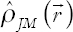of a nucleus .

Where, J is the multipolarity of the operator, M is the projection quantum number takes 2J + 1 values, −JMJ, YJM (Ωk) represents the spherical Harmonic, and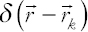is a Dirac delta function.

The matrix element in the reduced form of the operator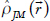is gotten when the transition happens from initial nuclear spin Ji to the final nuclear spin Jf and complying the inequality JiJJf , from Equation (4) where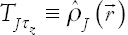then it is given by:

For the ground state (J = 0) as mentioned before. Moreover, Ji= Jf, and the charge density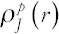define of the nucleus is getting from this matrix element .

The single-particle matrix element in Equation (4) can be represented by the radial wave functions of HO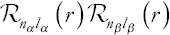and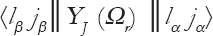which is the spherical Harmonic reduced matrix element.

After putting Equation (14) in Equation (13), the nuclear charge density becomes :

For the ground-state nucleus, (τz=1, -1), it makes J = 0 and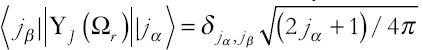. After utilizing the Delta-Kronecker in Equation (15) and putting τz= 1 for protons, the equation is rewritten as:

the index α≡nα lα jα used for all closed shells for the ground state.

The ground-state charge radii and charge distribution considered as two great determinable quantities experimentally, meanwhile, they can be calculated theoretically. The mean square radius for the nucleus gets from the charge density integration in Equation (16) .

Under the effect of the point-proton folded charge density distribution, in Equation (10), the charge density needs to be corrected by the folding factor .

Now, for the normalized charge density with the target nucleus atomic number Z, the root-mean-square in Equation (17) gives :

### 3. RESULTS AND DISCUSSION

The Beryllium nucleus has 12 known isotopes, but only one of these isotopes (Be9) is stable and a primordial nuclide. The microscopic structure of the stable nucleus Be9 imagined as being composed of a tightly bound core He4 plus five loosely bound nucleons outside the core divided over the p-shell (1 p3/2, 1 p1/2). On the other way, it consists of four protons and five neutrons.

In this paper, the CP effects are calculated according to Equation (7) which include M3Y and MSDI interactions.

The potential parameters of M3Y which known as three range potential contain spin orbit, central, and tensor interactions are obtained from Bertsch et al. . Besides, the MSDI strength parameters that used in the calculations of the CP effects are AT, B, and C. Where T is defined as the isospin (1, 0). They have taken the values as A0 =A1=B=25/A and C=0 , where A is the mass number of Beryllium nucleus, B and C are the correction parameters. It has the HO length parameter b = 1.791 fm .

FORTRAN 2008 used as a computer program for calculating CPM3Y and MSDI in the elastic and inelastic form factors. In addition, the OBDM elements calculated with the shell model code OXBASH for excitation states but is obtained from the occupation numbers for the closed-shell orbits (ground state).

Beryllium nucleus has a ground state, whereas its value is (Ji Ti = 3/2 1/2) E = 0.0 MeV. Here, two transitions are under investigation representing C2 with E = 2.43 MeV where the transition occurs to the excited state (Jf Tf = 5/2 1/2) and the other excited state is (Jf Tf = 7/2 1/2) E = 6.38 MeV o. In all graphs, the form factors with MS and CP effects including the realistic (M3Y) interactions representing as the red lines, the form factors with MSDI interaction performs as blue lines and the small filled circles represent the experimental values for the electron scattering form factors.

### 3.1. Elastic Coulomb form Factor for 3/2 −1/2 State

For elastic electron scattering, the scattered electron leaves the nucleus in the ground-state configuration. The ground state has (Jπ T= 3/2 1/2) with E = 0.0 MeV. The multipoles entering the elastic scattering are J = 0, 2 with the corresponding Coulomb transition C0 and C2, respectively. The calculated form factor of sum C0 + C2 is shown in Fig. 1.

Fig. 1. Elastic Coulomb C0+C2 form factors for Be9. The experimental data were taken from reference .

The obtained OBDM elements are shown in Table 1. The calculated root-mean-square (charge radii) for the ground state without folding is 2.629 fm but with folding is 2.505 fm, while experimentally is equal to 2.519 fm . The results with M3Y interaction have a great agreement with measured data in the transfer momentum domain of 1.1 ≤ q ≤2.5 fm−1. On the contrary, the calculations with MSDI interaction have a bad deal with the experimental data excepting the area of 1.5 ≤ q ≤ 2 fm−1 where they have similarities with each other.

TABLE 1 The calculated OBDM elements for the Coulomb C0+C2 transition of Be9

### 3.2. Inelastic Coulomb form Factor for 5/2 −1/2 State

The C2 transition for Coulomb scattering is taking place between the ground state of (Jπ T = 3/2 1/2) and the first excited state (Jπ T = 5/2 1/2) with excitation energy of E = 2.43 MeV. The computed and measured Coulomb form factors of inelastic electron scattering for the Be9 nucleus are shown in Fig. 2. The OBDM elements which calculated with OXBASH code are listed in Table 2. In this transition, the calculations with MSDI are not able to denote an adequate description of the experimental data for the region of transfer momenta (q = 0.8 fm−1) and (q = 1.8 fm−1), but once the CP effect with M3Y interaction is applied, making the results of the total theoretical form factors fitting the experimental data along with all regions of transfer momenta.

Fig. 2. Inelastic Coulomb C2 form factors for 5/2 1/2 state of Be9 with E = 2.43 MeV. The experimental data were taken from reference .

TABLE 2 The calculated OBDM elements for the Coulomb C2 transition of Be9

### 3.3. Inelastic Coulomb form Factor for 7/2 −1/2 State

The squared inelastic scattering of Coulomb form factors for Be9 is displayed in Fig. 3. The symbol of this transition (Coulomb transition) CJ = C2, it occurs between the ground state (Jπ T= 3/2 1/2) and the second excited state (Jπ T= 7/2 1/2) with transition energy E = 6.38 MeV. The OBDM elements are tabulated in Table 3. Fig. 3 shows the plot of measured and calculated data for the squared inelastic Coulomb scattering form factors. The ratio of agreement between the results of both M3Y and MSDI interactions for the Be9 form factors and the measured data are quite strong between (q = 1 fm−1) and (q = 2.5 fm−1). Taking into consideration that the form factors for the second excited state (Jπ T = 7/2 1/2) are not substantially different from that of the first excited state (Jπ T = 5/2 1/2), whence a noticeable change is observed in the computation of form factors by M3Y and MSDI interactions. The MSDI decreased faster than the M3Y during the increase of momentum transfer, especially at the point of (q = 3 fm−1).

Fig. 3. Inelastic Coulomb C2 form factors for 7/2 1/2 state of Be9 with E = 6.38 MeV. The experimental data were taken from reference .

TABLE 3 The calculated OBDM elements for the Coulomb C2 transition of Be9

### 4. CONCLUSION

In the present work, it is possible to consider the following conclusions:

• • The basic calculations include the Coulomb form factors for the ground state and other excitation states.

• • The ground-state Coulomb form factors (C0 transitions) for the M3Y interaction and the ground-state charge radii with folding effect give the best fit with the experimental data for beryllium (Be9) nucleus.

• • For Be9 nucleus which is under consideration, the quality of similarity between the computed Coulomb form factors FC(q) and those of the measured data become even better in the using of the CP effects with including M3Y residual interaction for all Coulomb C2 transitions because M3Y interaction is more realistic nucleon-nucleon interaction that adopted for the CP calculation.

• • The calculation of Coulomb form factors FC(q) with (MSDI) interaction is dealing with the surface nucleons only. Therefore, it has a limited agreement with the experimental results.

• • The HO succeeded to describe the wave functions completely.

### 5. CONFLICTS OF INTEREST

There are no conflicts of interest.

### REFERENCES

. T. W. Donnelly and J. D. Walecka. “Electron scattering and nuclear structure“. Annual Review of Nuclear Science, vol. 25, no. 1, pp. 329-405, 1975.

. D. J. Millener, D. I. Sober, H. Crannell, J. T. O'Brien, L. W. Fagg and L. Lapikas. “Inelastic electron scattering from 13C“. Physical Reviews, vol. 39, no. 1, pp.14-46, 1989.

. S. Cohen and D. Kurath. “Effective interactions for the 1p shell“. Nuclear Physics, vol. 73, no. 1, pp. 1-24, 1965.

. D. Salman, S. A. Al-Ramahi and M. H. Oleiwi. “Inelastic electron-nucleus scattering form factors for 64, 66, 68Zn isotopes“. Vol. 2144. In: AIP Conference Proceedings, 030029, 2019.

. K. S. Jassim. “Longitudinal form factor for some sd-shell nuclei using large scale model space“. International Journal of Science and Technology, vol. 1, no. 3, pp. 140-143, 2011.

. N. F. Mott. “Sir Nevill Mott:65 Years in Physics“.Vol. 12. World Scientific, Singapore, 1995.

. Z. Czyżewski, D. O. N. MacCallum, A. Romig and D. C. Joy. “Calculations of Mott scattering cross section“. Journal of Applied Physics, vol. 68, no. 7, pp. 3066-3072, 1990.

. H. Uberall. “Electron Scattering from Complex Nuclei V36A“. Academic Press, New York, London, 2012.

. F. A. Majeed. “The effect of core polarization on longitudinal form factors in 10B“. Physica Scripta, vol. 85, no. 6, 065201, 2012.

. G. Fricke, C. Bernhardt, K. Heilig, L. A. Schaller, L. Schellenberg, E. B. Shera and C. W. Dejager. “Nuclear ground state charge radii from electromagnetic interactions“. Atomic Data and Nuclear Data Tables, vol. 60, no. 2, pp. 177-285, 1995.

. F. I. Sharrad, A. K. Hamoudi, R. A. Radhi, H. Y. Abdullah, A. A. Okhunov and H. A. Kassim. “Elastic electron scattering from some light nuclei“. Chinese Journal of Physics, vol. 51, no. 3, pp. 452-465, 2013.

. R. A. Radhi, A. A. Alzubadi and E. M. Rashed. “Shell model calculations of inelastic electron scattering for positive and negative parity states in 19F“. Nuclear Physics A, vol. 947, pp. 12-25, 2016.

. E. M. Raheem, R. O. Kadhim and N. A. Salman. “The effects of core polarization on some even-even sd-shell nuclei using Michigan three-range Yukawa and modified surface delta interactions“. Pramana, vol. 92, no. 3, 39, 2019.

. B. A. Brown, A. Etchegoyen, N. S. Godwin, W. D. M. Rae, W. A. Richter, W. E. Ormand and C. H. Zimmerman. “Oxbash for windows PC“. In:MSU-NSCL Report, pp. 1289, 2004.

. S. Mohammadi, B. N. Giv and N. S. Shakib. “Energy levels calculations of 24Al and 25Al isotopes“. Nuclear Science, vol. 2, no. 1, pp. 1-4, 2017.

. T. de Forest and J. D. Walecka. “Electron scattering and nuclear structure“. Advances in Physics, vol. 15, no. 57, pp. 1-109, 1966.

. L. J. Tassie and F. C. Barker. “Application to electron scattering of center-of-mass effects in the nuclear shell model“. Physical Review, vol. 111, no. 3, 940, 1958.

. K. S. Jassim, A. A. Al-sammarrae, F. I. Sharrad and H. A. Kassim. “Elastic and inelastic electron-nucleus scattering form factors of some light nuclei:Na 23, Mg 25, Al 27, and Ca 41“. Physical Review C, vol. 89, no. 1, 014304, 2014.

. H. Fatah, R. A. Radhi and N. R. Abdullah. “Analytical derivations of single-particle matrix elements in nuclear shell model“. Communications in Theoretical Physics, vol. 66, no. 1, 104, 2016.

. P. J. Brussaard and W. M. Glaudemans. “Shell-Model Application in Nuclear Spectroscopy“.North-Holland, Amsterdam, 1977.

. D. Salman, D. R. Kadhim. “Longitudinal electron scattering form factors for 54, 56 Fe“. International Journal of Modern Physics E, vol. 23, no. 10, 1450054, 2014.

. F. I. Sharrad, A. K. Hamoudi, R. A. Radhi, Y. Abdullah, A. A. Okhunov and H. A. Kassim. “Elastic electron scattering from some light nuclei“. Chinese Journal of Physics, vol. 51, no. 3, pp. 452-465, 2013.

. B. A. Brown, R. Radhi and B. H. Wildenthal. “Electric quadrupole and hexadecupole nuclear excitations from the perspectives of electron scattering and modern shell-model theory“. Physics Reports, vol. 101, no. 5, pp. 313-358, 1983.

. H. M. Dlshad and A. H. R. Fatah. “Using MSDI and M3Y core polarization for the coulomb electron scattering for some ground state nuclei“. JZS (Part-A), vol. 21, no. 2, pp. 11-20, 2019.

. G. S. Anagnostatos, A. N. Antonov, P. Ginis, J. Giapitzakis and M. K. Gaidarov. “On the central depression in density of“. Journal of Physics G:Nuclear and Particle Physics, vol. 25, no. 1, 69, 1999.

. G. Bertsch, J. Borysowicz, H. McManus and W. G. Love. “Interactions for inelastic scattering derived from realistic potentials“. Nuclear Physics A, vol. 284, no. 3, pp. 399-419, 1977.

. F. A. Majeed. “Longitudinal and transverse form factors from 12C“. Physica Scripta, vol. 76, no. 4, 332, 2007.

. J. P. Glickman, W. Bertozzi, T. N. Buti, S. Dixit, F. W. Hersman, C. E. Hyde-Wright and B. L. Berman. “Electron scattering from sup 9 be“. Physical Review C (Nuclear Physics) (USA), vol. 43, no. 4, pp. 1740-1757, 1991.

. Angeli and K. P. Marinova. “Table of experimental nuclear ground state charge radii:An update“. Atomic Data and Nuclear Data Tables, vol. 99, no. 1, pp. 69-95, 2013.